# DAV Class 5 Maths Chapter 4 Brain Teasers Solutions

The DAV Class 5 Maths Book Solutions Pdf and DAV Class 5 Maths Chapter 4 Brain Teasers Solutions of Fractional Numbers offer comprehensive answers to textbook questions.

## DAV Class 5 Maths Ch 4 Brain Teasers Solutions

Question 1.
(a) $$\frac{1}{3}-\frac{1}{9}$$ is
(i) $$\frac{1}{9}$$
(ii) $$\frac{2}{9}$$
(iii) $$\frac{1}{3}$$
(iv) $$\frac{1}{2}$$
Solution:
$$\frac{1}{3}-\frac{1}{9}$$
LCM = 9
$$\frac{1}{3}-\frac{1}{9}$$ = $$\frac{3}{9}-\frac{1}{9}$$
= $$\frac{3-1}{9}$$
= $$\frac{2}{9}$$
So option (ii) is correct.

(b) A fraction is greater than one, if-
(i) Numerator = Denominator
(ii) Numerator < Denominator (iii) Numerator > Denominator
(iv) Numerator = 1
Solution:
Numerator > Denominator
Option (iii) is correct.

(c) $$\frac{2}{3}$$ of an hour = _____ minutes.
(i) 40 minutes
(ii) 50 minutes
(iii) 60 minutes
(iv) 20 minutes
Solution:
$$\frac{2}{3}$$ of hour
1 hour = 60 minutes.
$$\frac{2}{3}$$ of hour = $$\frac{2}{3}$$ × 60 = 40 minutes.
So option (i) is correct.

(d) What number should be added to $$\frac{8}{9}$$ to get $$\frac{9}{8}$$?
(i) $$\frac{9}{8}$$
(ii) 1
(iii) $$\frac{17}{72}$$
(iv) $$\frac{19}{72}$$
Solution:
$$\frac{9}{8}-\frac{8}{9}$$
(First convert the fractions into like fractions).
LCM = 72
$$\frac{9}{8}-\frac{8}{9}$$ = $$\frac{81}{72}-\frac{64}{72}$$ = $$\frac{17}{72}$$
So option (iii) is correct.

(e) What fraction is the shaded portion?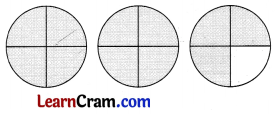(i) 2
(ii) 1$$\frac{1}{2}$$
(iii) 1$$\frac{3}{4}$$
(iv) 2$$\frac{3}{4}$$
Solution: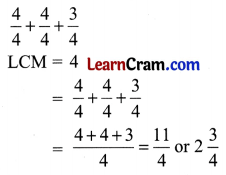So option (iv) is correct.

Question 2.
Find the sum:
(a) 7 + 1$$\frac{1}{2}$$ + $$\frac{9}{5}$$
Solution:
7 + 1$$\frac{1}{2}$$ + $$\frac{9}{5}$$ = $$\frac{7}{1}+\frac{3}{2}+\frac{9}{5}$$
(First convert into like fraction by finding LCM)
LCM of 1, 2 and 5 = 10
$$\frac{7}{1}+\frac{3}{2}+\frac{9}{5}$$ = $$\frac{70}{10}+\frac{15}{10}+\frac{18}{10}$$
= $$\frac{70+15+18}{10}$$
= $$\frac{103}{10}$$
= 10$$\frac{3}{10}$$

(b) 2$$\frac{1}{2}$$ + 1$$\frac{1}{4}$$ + 2$$\frac{4}{5}$$
Solution:
2$$\frac{1}{2}$$ + 1$$\frac{1}{4}$$ + 2$$\frac{4}{5}$$ = $$\frac{5}{2}+\frac{5}{4}+\frac{14}{5}$$
First convert into like fraction by finding LCM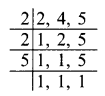LCM of 2, 4 and 5 = 20
$$\frac{5}{2}+\frac{5}{4}+\frac{14}{5}$$ = $$\frac{50}{20}+\frac{25}{20}+\frac{56}{20}$$
= $$\frac{50+25+56}{20}$$
= $$\frac{131}{20}$$
= 6$$\frac{11}{20}$$

Question 3.
Subtract.
(a) 4$$\frac{1}{2}$$ from 6
Solution:
6 – 4$$\frac{1}{2}$$ = $$\frac{6}{1}-\frac{9}{2}$$ (convert into like fractions)
LCM of 1 and 2 = 2
$$\frac{6}{1}-\frac{9}{2}$$ = $$\frac{12}{2}-\frac{9}{2}$$
= $$\frac{3}{2}$$
= 1$$\frac{1}{2}$$

(b) 2$$\frac{1}{2}$$ from 7$$\frac{3}{5}$$
Solution:Question 4.
Find the value of $$2 \frac{3}{5}+3 \frac{1}{2}-2 \frac{1}{8}$$
Solution: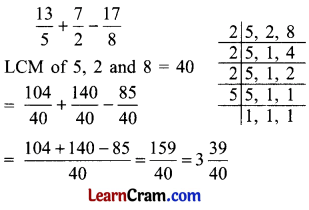Question 5.
Sheetal needs 1$$\frac{1}{2}$$ metres red ribbon, $$\frac{3}{4}$$ metres yellow ribbon and one metre black ribbon to make a doll. Find the total length of ribbon needed.
Solution:
Length of red ribbon = 1$$\frac{1}{2}$$ m = $$\frac{3}{2}$$ m.
Length of yellow ribbon = $$\frac{3}{4}$$ m.
Length of black ribbon = 1 m.
Total length of ribbon = $$\frac{3}{2}+\frac{3}{4}+\frac{1}{1}$$
= $$\frac{6+3+4}{4}$$
= $$\frac{13}{4}$$
= 3$$\frac{1}{4}$$
Total length of ribbon needed is 3$$\frac{1}{4}$$ m.

Question 6.
Renu’s mother bought five litres of milk, 2$$\frac{1}{2}$$ litres milk was used for making sweets, $$\frac{3}{4}$$ litres for making tea. How many litres of milk is left?
Solution:
Total quality of milk = 5 l
Quantity of milk used in making sweets = 2$$\frac{1}{2}$$ = $$\frac{5}{2}$$ l
Milk used for making tea = $$\frac{3}{4}$$ l
Total quantity of milk used = $$\frac{5}{2}+\frac{3}{4}$$
LCM of 2 and 4 = 4
$$\frac{5}{2}+\frac{3}{4}$$ = $$\frac{10+3}{4}$$ = $$\frac{13}{4}$$ l
Milk left = $$\frac{5}{1}-\frac{13}{4}$$
LCM of 1 and 4 = 4
$$\frac{5}{1}-\frac{13}{4}$$ = $$\frac{20}{4}-\frac{13}{4}$$
= $$\frac{7}{4}$$
= 1$$\frac{3}{4}$$ l
1$$\frac{3}{4}$$ litre of milk is left.

Question 7.
Reduce into lowest terms.
(a) $$\frac{8}{12}$$
Solution:
$$\frac{8}{12}=\frac{4}{6}=\frac{2}{3}$$

(b) $$\frac{35}{63}$$
Solution:
$$\frac{35}{63}=\frac{5}{9}$$

(c) $$\frac{44}{99}$$
Solution:
$$\frac{44}{99}=\frac{4}{9}$$

(d) $$\frac{6}{10}$$
Solution:
$$\frac{6}{10}=\frac{3}{5}$$

Question 8.
Arrange in ascending order.
(a) $$\frac{3}{4}, \frac{7}{10}, \frac{1}{2}, \frac{5}{8}$$
Solution:(b) $$1 \frac{5}{6}, \frac{11}{9}, \frac{5}{16}, 3$$
Solution:Question 9.
Find the product.
(a) $$\frac{4}{12} \times \frac{21}{18} \times \frac{35}{25}$$
Solution: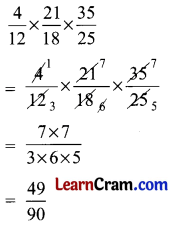(b) $$1 \frac{1}{4} \times 2 \frac{3}{5} \times 2 \frac{4}{5}$$
Solution: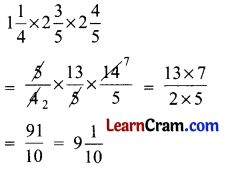Question 10.
Solve these division sums.
(a) $$9 \frac{5}{8} \div 2 \frac{1}{4}$$
Solution: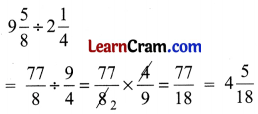(b) 52 ÷ 2$$\frac{3}{5}$$
Solution:Question 11.
State which of the following statements are true.
(а) The reciprocal of $$\frac{9}{7}$$ is 1$$\frac{2}{7}$$.
Solution:
False
Explanation: The reciprocal of $$\frac{9}{7}$$ is $$\frac{7}{9}$$

(b) The multiplicative inverse of 1 is 1.
Solution:
True

(c) $$\frac{1}{2} \div \frac{1}{4}$$ means how many quarters in $$\frac{1}{2}$$.
Solution:
True

(d) The product of fractional number and one is one.
Solution:
False
Explanation: fractional number × 1 = fractional number

(e) $$\frac{4}{9} \div \frac{4}{9}=\frac{81}{16}$$
Solution:
False
Explanation: $$\frac{4}{9} \div \frac{4}{9}=1$$

(f) $$0 \div \frac{2}{3}=\frac{2}{3}$$
Solution:
False
Explanation: 0 ÷ $$\frac{2}{3}$$ = 0

Question 12.
A family consumes 2$$\frac{1}{2}$$ litres of milk every day. What is the total consumption of milk by the family in the month of April?
Solution:
Consumption of milk in one day = 2$$\frac{1}{2}$$ l
Consumption of milk in the month of April (30 days) = $$\frac{5}{2}$$ × 30 = 75
Total consumption of milk by the family in the month of April is 75 litres.

Question 13.
Mala has 36 toffees. She gives $$\frac{4}{9}$$ of them to her friend. How many toffees are left with her?
Solution:
Total no. of toffees = 36
No. of toffees given = $$\frac{4}{9}$$ of 36
Toffees left = $$\frac{4}{9}$$ × 36 = 16
16 toffees are left with her.

Question 14.
If the shaded portion has a value of $$\frac{1}{3}$$, what is the value of the whole shape?Solution:
Let 1 small triangle show $$\frac{1}{3}$$ part.
In above fig. there are 5 rectangles which makes 5 × 2 = 10 triangles.
3 more triangles are also present.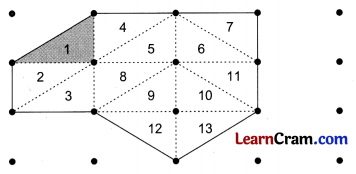Total value of whole shape = 13 × $$\frac{1}{3}$$
= $$\frac{13}{3}$$
= 4$$\frac{1}{3}$$

Question 1.
Write the next four equivalent fractions.
(a) $$\frac{4}{7}, \frac{8}{14}, \frac{12}{21}$$, ____, _____, _____, _____
Solution:(b) $$\frac{2}{3}, \frac{4}{6}, \frac{6}{9}$$, ____, _____, _____, _____
Solution: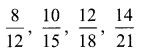(c) $$\frac{4}{5}, \frac{8}{10}, \frac{12}{15}$$, ____, _____, _____, _____
Solution:Question 2.
Find the missing numerals.
(a)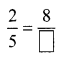Solution: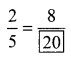(b)Solution: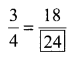(c)Solution:(d)Solution: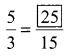(e)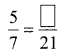Solution: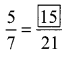Question 3.
Compare the fractions by cross multiplication method.
(a) $$\frac{2}{7}$$ and $$\frac{2}{8}$$
Solution:
$$\frac{2}{7}$$ and $$\frac{2}{8}$$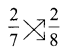2 × 8 = 16
7 × 2 = 14
$$\frac{2}{7}>\frac{2}{8}$$ (because 16 > 14)

(b) $$\frac{3}{10}$$ and $$\frac{2}{5}$$
Solution:
$$\frac{3}{10}$$ and $$\frac{2}{5}$$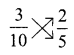3 × 5 = 15
10 × 2 = 20
$$\frac{3}{10}<\frac{2}{5}$$ (because 15 < 20)

(c) $$\frac{4}{9}$$ and $$\frac{5}{18}$$
Solution:
$$\frac{4}{9}$$ and $$\frac{5}{18}$$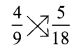4 × 18 = 72
9 × 5 = 45
$$\frac{4}{9}>\frac{5}{18}$$ (because 72 > 45)

(d) $$\frac{9}{19}$$ and $$\frac{9}{10}$$
Solution:
$$\frac{9}{19}$$ and $$\frac{9}{10}$$9 × 10 = 90
19 × 9 = 171
$$\frac{9}{19}<\frac{9}{10}$$ (because 90 < 171)

Question 4.
Arrange the following in ascending order.
(a) $$\frac{2}{5}, \frac{2}{3}, \frac{7}{5}, \frac{11}{20}$$
Solution: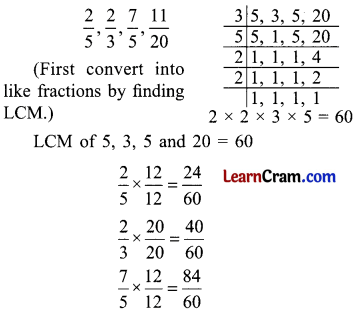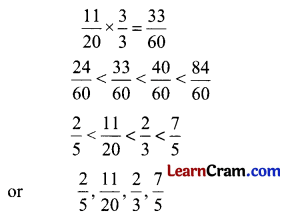(b) $$1 \frac{1}{2}, \frac{9}{20}, \frac{13}{10}, \frac{8}{15}$$
Solution: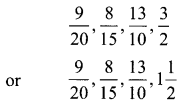Question 5.
Multiply.
(a) 70 × $$\frac{14}{5}$$
Solution: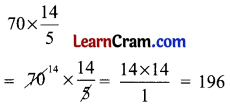(b) 105 × 1$$\frac{1}{5}$$
Solution:(c) 100 × 3$$\frac{1}{10}$$
Solution: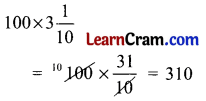(d) 45 × 3$$\frac{1}{9}$$
Solution:(e) 52 × $$\frac{3}{13}$$
Solution: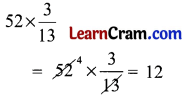Question 6.
Find the reciprocal of the following:
(a) 2$$\frac{1}{7}$$
Solution:
2$$\frac{1}{7}$$ = $$\frac{15}{7}$$
Reciprocal of $$\frac{15}{7}$$ = $$\frac{7}{15}$$

(b) 8
Solution:
Reciprocal of 8 = $$\frac{1}{8}$$

(c) $$\frac{5}{12}$$
Solution:
Reciprocal of $$\frac{5}{12}$$ = $$\frac{12}{5}$$

(d) 5$$\frac{1}{3}$$
Solution:
5$$\frac{1}{3}$$ = $$\frac{16}{3}$$
Reciprocal of $$\frac{16}{3}$$ = $$\frac{3}{16}$$

(e) 1$$\frac{3}{7}$$
Solution:
1$$\frac{3}{7}$$ = $$\frac{10}{7}$$
Reciprocal of $$\frac{10}{7}$$ = $$\frac{7}{10}$$

Question 7.
Arrange in ascending order:
(a) $$\frac{5}{6}, \frac{13}{3}, \frac{7}{18}, \frac{5}{8}$$
Solution:
$$\frac{7}{18}, \frac{5}{8}, \frac{5}{6}, \frac{13}{3}$$

(b) $$\frac{5}{6}, \frac{7}{5}, 2, \frac{5}{16}$$
Solution:
$$\frac{5}{16}, \frac{5}{6}, \frac{7}{5}, 2$$

Question 8.
(a)Solution:
1$$\frac{1}{4}$$

(b)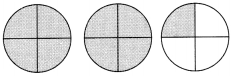Solution:
2$$\frac{1}{4}$$

Question 9.
State true or false:
(a) The product of fractional number and 0 is fractional number.
Solution:
False

(b) The reciprocal of $$\frac{2}{3}$$ is $$\frac{3}{1}$$.
Solution:
False

(c) $$\frac{1}{3} \div \frac{1}{4}$$ means how many quarter in $$\frac{1}{3}$$.
Solution:
True

(d) $$\frac{3}{7} \div \frac{2}{7}=\frac{3}{2}$$
Solution:
True